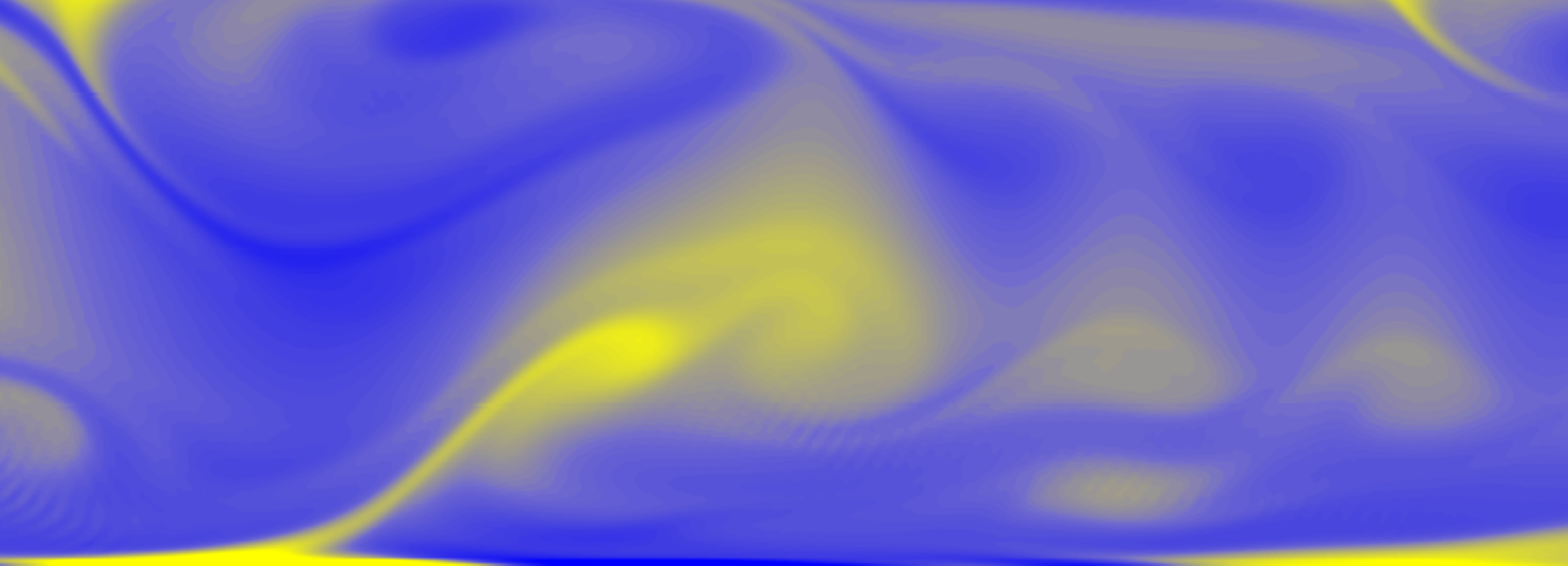## SFMC23## On the purely inertial collapse of gas cavities: Bubble bursting jets

• Gordillo Arias de Saavedra, José Manuel (Universidad de Sevilla)
• Blanco Rodríguez, Francisco José (Universidad de Sevilla)

Our numerical simulations reveal that the jets originated from the bursting of bubbles are driven by the condition that the dimensionless liquid flow rate per unit length directed towards the axis of symmetry, $q_\infty$, remains nearly constant in time. This is so because liquid inertia prevents appreciable changes in $q_\infty$ during the short time scale characterizing the jet ejection process, which justify the purely inertial scalings for the jet width and velocity found here, $r_{jet}\propto\sqrt{q_\infty\tau}$ and $v_{jet}\propto \sqrt{q_\infty/\tau}$, with $\tau$ indicating the dimensionless time after the jet is ejected, a result which notably differs from the common belief that the jet width and velocity follow the inertio-capillary scaling $r_{jet}\propto \tau^{2/3}$ and $v_{jet}\propto \tau^{-1/3}$. Our physical description, which neglects capillary forces, is in close agreement with numerical simulations from the instant of jet inception until the jet width is comparable to that of the initial bubble.